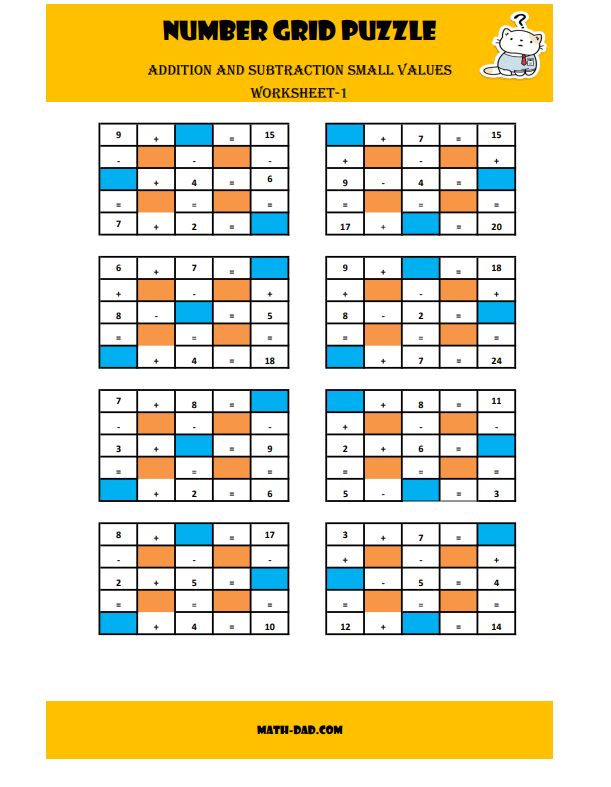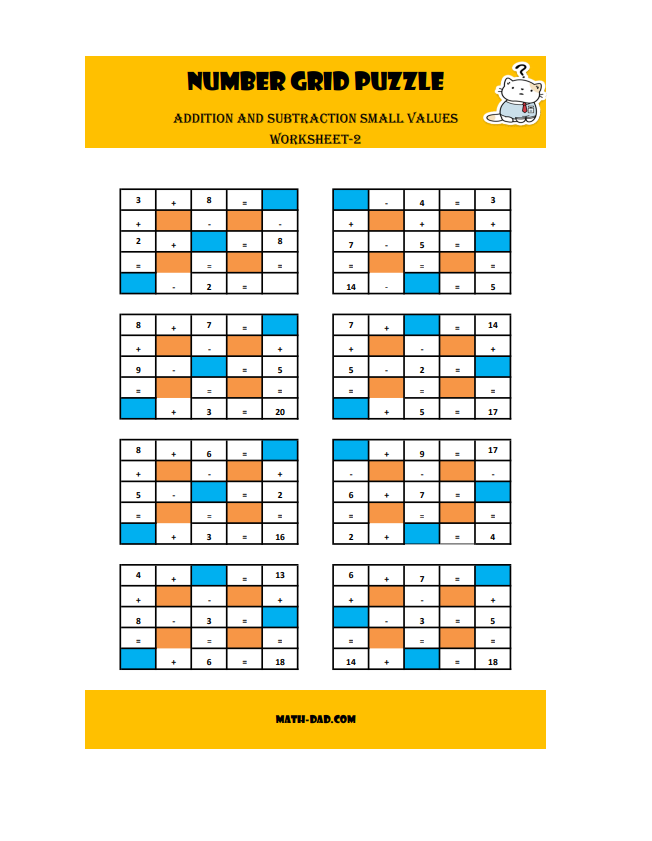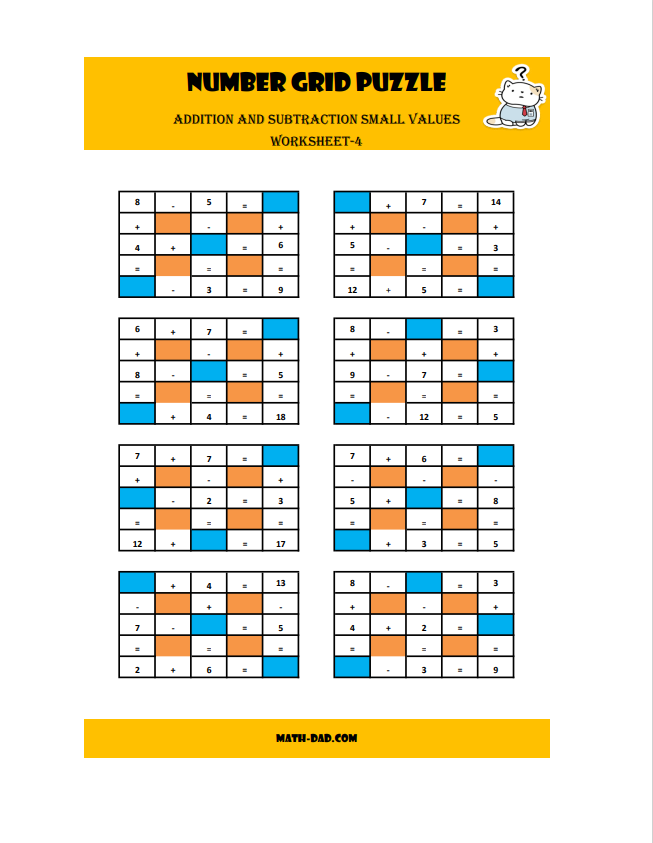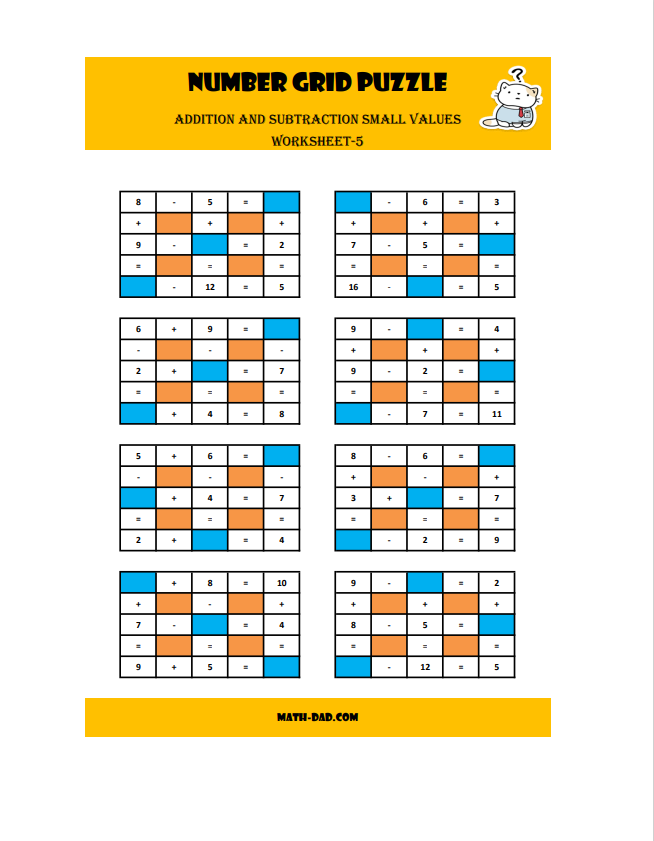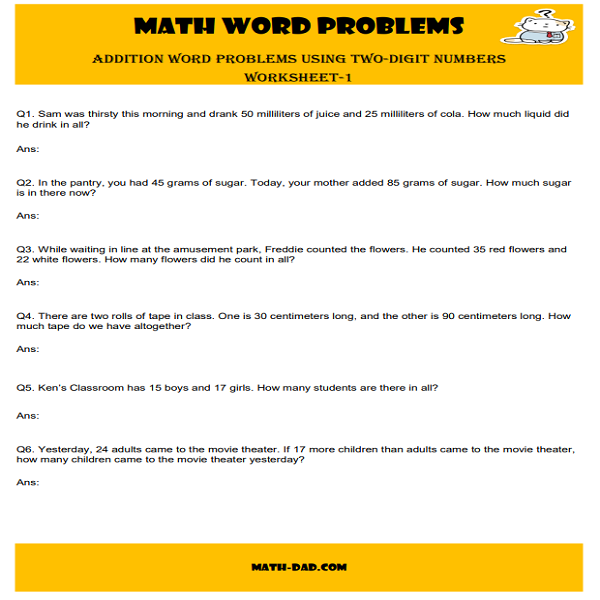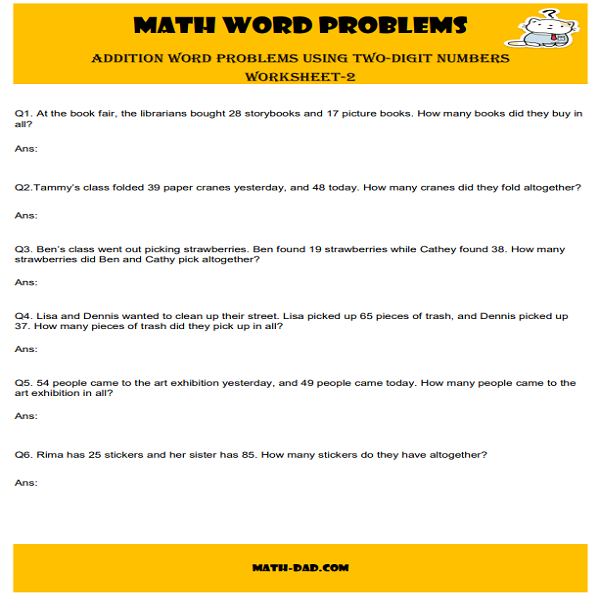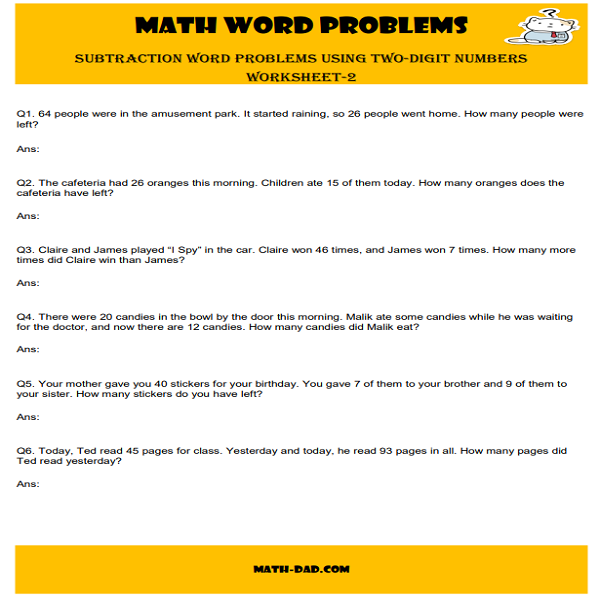# Math Worksheets

Our math worksheets based on math facts, one digit and two-digit addition, subtraction, multiplication, and division. All the Worksheets designed based on the online activities from our website is printable. Ideal for students from Grade-1 to Grade-3. Practicing math facts help students to sharpen their math skills, develop independent thinking and simulate creativity.# SubtractionOur subtraction worksheets based on subtraction facts, one digit and two digit-subtraction is printable. Learn more...

# Multiplication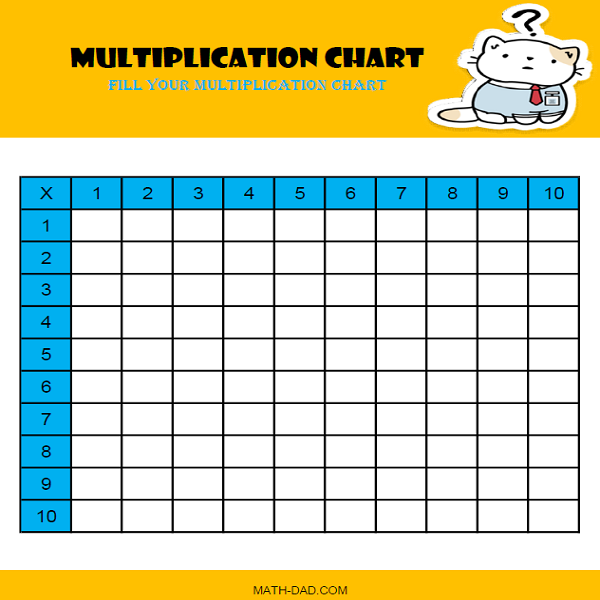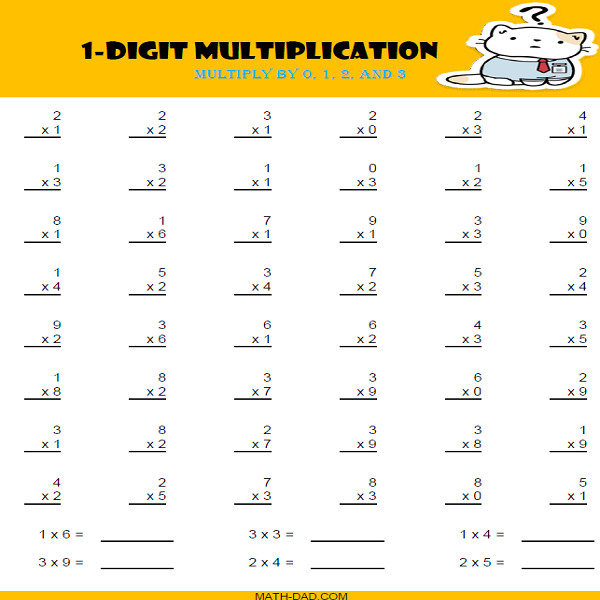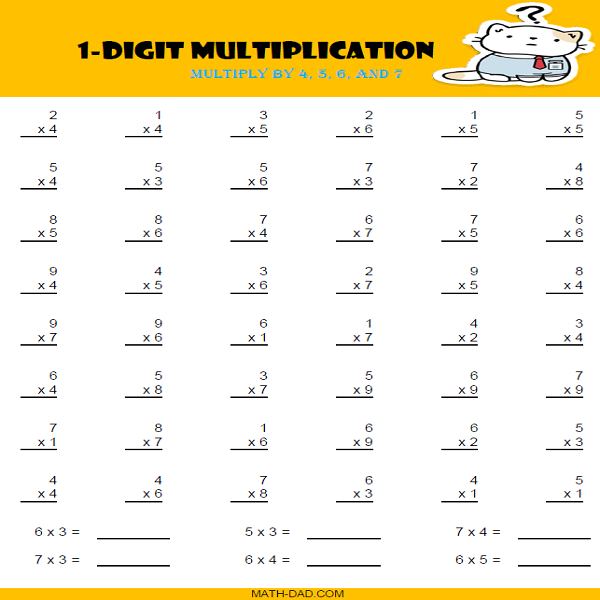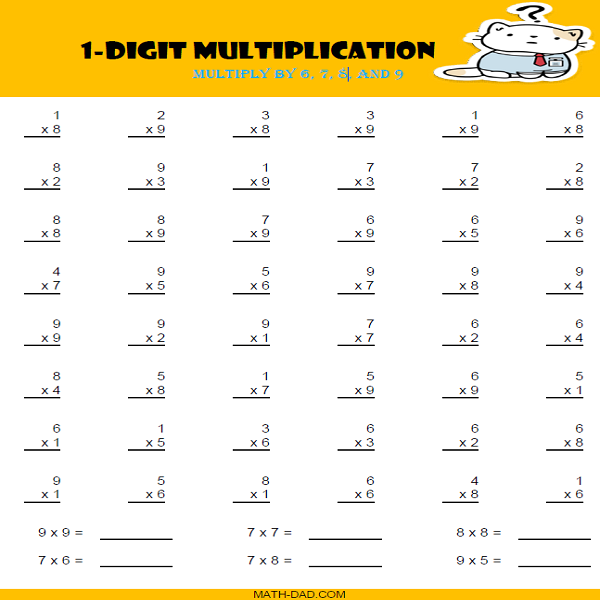Our multiplication worksheets based on multiplication facts, one digit and two-digit multiplication is printable. Learn more...

# Division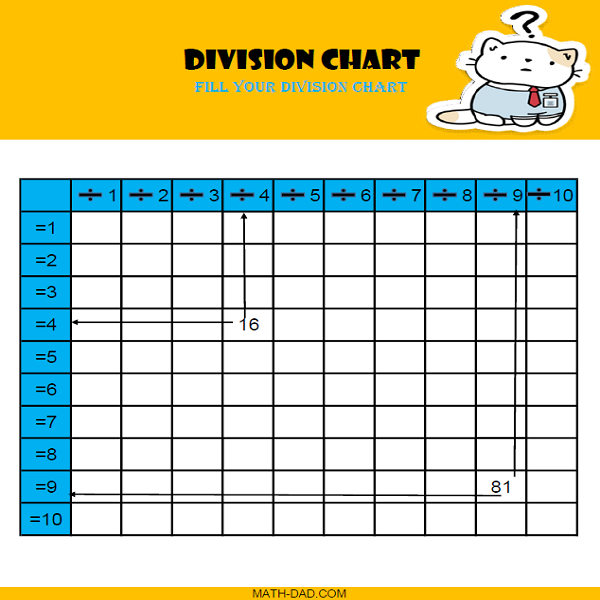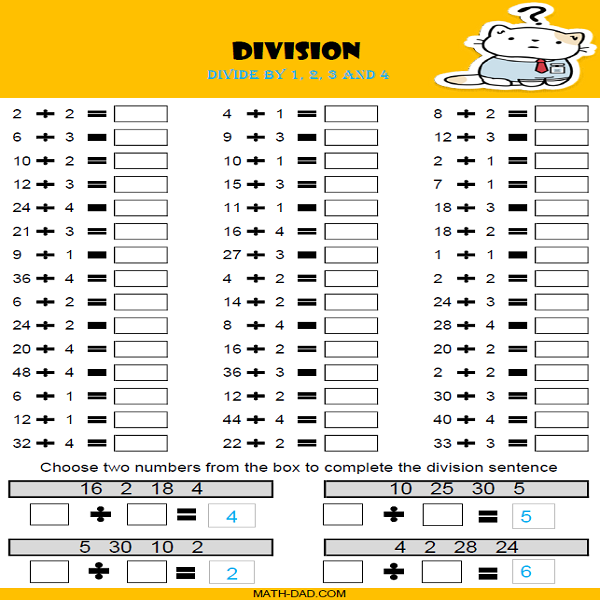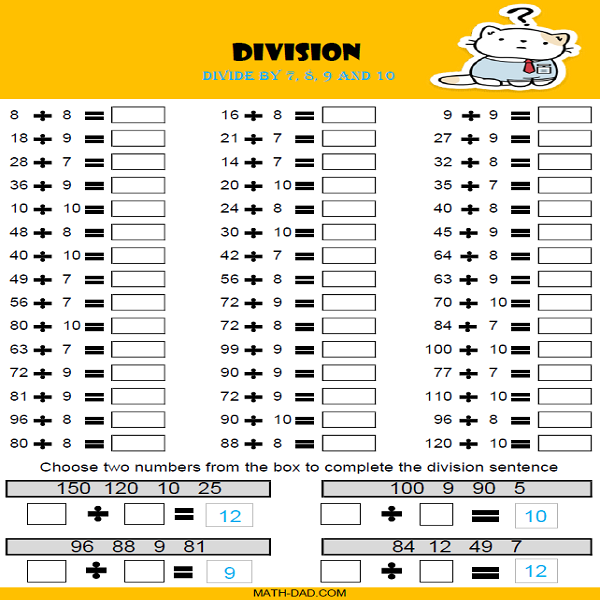Our division worksheets based on division facts, one digit and two-digit division is printable. Learn more...

# Number Grid Puzzles